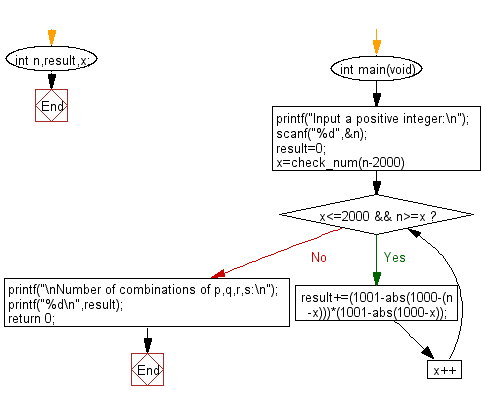﻿ C : Number of combinations that satisfy p + q + r + s = n

# C Exercises: Find the number of combinations that satisfy p + q + r + s = n

## C Basic Declarations and Expressions: Exercise-147 with Solution

Write a C program to find the number of combinations that satisfy p + q + r + s = n where n is a given number <= 4000 and p, q, r, s are between 0 and 1000.

Sample Solution:

C Code:

``````#include <stdio.h>
#include <stdlib.h>

// Define a macro to ensure non-negative numbers
#define check_num(x) (x>0?x:0)

// Define global variables
int n, result, x;

int main(void) {
// Prompt user for input
printf("Input a positive integer:\n");

scanf("%d", &n);

// Initialize result variable
result = 0;

// Loop through possible values of x within the specified range
for (x = check_num(n - 2000); x <= 2000 && n >= x; x++) {
// Calculate the result based on the given formula
result += (1001 - abs(1000 - (n - x))) * (1001 - abs(1000 - x));
}

// Print the number of combinations
printf("\nNumber of combinations of p,q,r,s:\n");
printf("%d\n", result);

// Return 0 to indicate successful execution
return 0;
}
``````

Sample Output:

```Input a positive integer:
25

Number of combinations of p,q,r,s:
3276
```

Flowchart:C programming Code Editor:

What is the difficulty level of this exercise?

Test your Programming skills with w3resource's quiz.

﻿